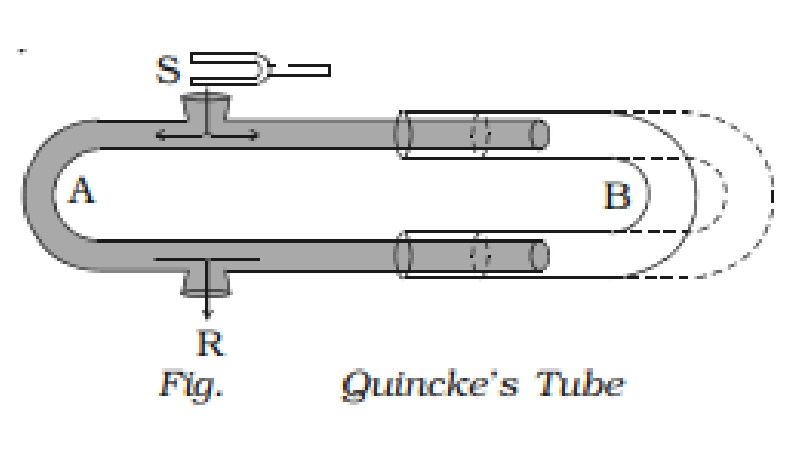Home | | Physics | | Physics | Experimental demonstration of interference of sound

# Experimental demonstration of interference of soundThe phenomenon of interference between two longitudinal waves in air can be demonstrated by Quincke?s tube shown in Fig..

Experimental demonstration of interference of sound

The phenomenon of interference between two longitudinal waves in air can be demonstrated by Quincke?s tube shown in Fig..

Quincke?s tube consists of U shaped glass tubes A and B. The tube SAR has two openings at S and R. The other tube B can slide over the tube A. A sound wave from S travels along both the paths SAR and SBR in opposite directions and meet at R.

If the path difference between the two waves (i.e) SAR ~ SBR is an integral multiple of wavelength, intensity of sound will be maximum due to constructive interference.

i.e SAR ~ SBR = mλ The corresponding phase difference φ between the two waves is even multiples of π. (i.e) φ = m 2π where m = 0, 1, 2, 3 ....

If the tube B is gradually slided over A, a stage is reached when the intensity of sound is zero at R due to destructive interference. Then no sound will be heard at R.If the path difference between the waves is odd multiples of λ/2 , intensity of sound will be minimum.

i.e.   SAR ~ SBR = (2m + 1) λ/2

The corresponding phase difference φ between the two waves is odd multiples of π. (i.e) φ = (2m + 1)π where m = 0, 1, 2, 3 ....

Study Material, Lecturing Notes, Assignment, Reference, Wiki description explanation, brief detail

Related Topics